# Four numbers

The first number is 50% second, the second number is 40% third, the third number is 20% of the fourth. The sum is 396. What are the numbers?

a =  12
b =  24
c =  60
d =  300

### Step-by-step explanation:

$b=24=24$
$c=60=60$
$d=300=300$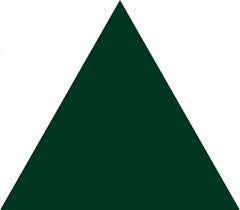Did you find an error or inaccuracy? Feel free to write us. Thank you!Tips to related online calculators
Do you have a linear equation or system of equations and looking for its solution? Or do you have a quadratic equation?

## Related math problems and questions:

• Sum of three numbersThe sum of three numbers from which the second number is 20% smaller than the first number and the third number is 25% smaller than the second number is 96. Determine these numbers.
• Geography testsOn three 150-point geography tests, you earned grades of 88%, 94%, and 90%. The final test is worth 250 points. What percent do you need on the final to earn 93% of the total points on all tests?
• The ratio 4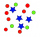The ratio of two number is 5:4 if 40% of the first number is 12, what will be 50% of the second number?
• PharmacyAt the pharmacy are in one container 20% solution in the second 50% solution of disinfectant. They need to prepare 4 L of 48-percent solution. What amount of solution from each container is needed to mix?
• Four pupilsFour pupils divided $1485 so that the second received 50% less than the first, the third 1/2 less than a fourth, and fourth$ 154 less than the first. How much money had each of them?
• RemaindersIt is given a set of numbers { 170; 244; 299; 333; 351; 391; 423; 644 }. Divide this numbers by number 66 and determine set of remainders. As result write sum of this remainders.
• 40% volume40% volume with 104 uph (units per labor hour) 8 people working. What is the volume?
• Alcohol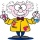6l 48% alcohol, how much 52% alcohol must be added to give 50% alcohol?
• Energy savingThey were released three different, independent inventions saving 20%, 24% and 15% energy. Some considered that while the use of these inventions, the total savings will be 20% + 24% + 15% = 59%. Is this true? How much will percent of energy save all thre
• The notebook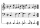After rising by 40%, the notebook cost 10.50 euros. How much did this notebook cost if it increased in price by only 20% instead of 40%?
• Acid solutionBy adding 250 grams of a 96% sulfuric acid solution to its 3% solution, we changed its initial concentration to 25%. How many grams of 3% of the acid was used for dilution?
• Cinema ticketsCinema sold 180 tickets this Thursday, which is 20%. Monday 14%, Tuesday 6%, Wednesday 9%, Friday 24%, Saturday 12%, and Sunday 15%. How many tickets were sold per week?
• Four-digit numbers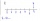Find four-digit numbers where all the digits are different. For numbers, the sum of the third and fourth digits is twice the sum of the first two digits, and the sum of the first and fourth digits is equal to the sum of the second and third digits. The di
• Three numbers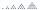What are three numbers that have the property: the sum of the first and second numbers' reciprocals is 12/7, the first and third 11/24, and the second and the third 3/8.
• Two numbersWe have two numbers. Their sum is 140. One-fifth of the first number is equal to half the second number. Determine those unknown numbers.
• Three workers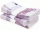The three workers received € 2,850 together for the work done. They divided them according to the time worked so that the first received 20% less than the second and the third € 50 more than the second. How much EUR did each worker receive?
• School year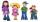At the end of the school year has awarded 20% of the 250 children who attend school. Award got 18% boys and 23% of girls. Find how many boys and how many girls attend school.